importONNXLayers

Import layers from ONNX network

Description

example

lgraph = importONNXLayers(modelfile) imports the layers and weights of a pretrained ONNX™ (Open Neural Network Exchange) network from the file modelfile. The function returns lgraph as a LayerGraph object compatible with a DAGNetwork or dlnetwork object.

importONNXLayers requires the Deep Learning Toolbox™ Converter for ONNX Model Format support package. If this support package is not installed, then importONNXLayers provides a download link.

Note

By default, importONNXLayers tries to generate a custom layer when the software cannot convert an ONNX operator into an equivalent built-in MATLAB® layer. For a list of operators for which the software supports conversion, see ONNX Operators Supported for Conversion into Built-In MATLAB Layers.

importONNXLayers saves the generated custom layers in the package +modelfile.

importONNXLayers does not automatically generate a custom layer for each ONNX operator that is not supported for conversion into a built-in MATLAB layer. For more information on how to handle unsupported layers, see Tips.

example

lgraph = importONNXLayers(modelfile,Name=Value) imports the layers and weights from an ONNX network with additional options specified by one or more name-value arguments. For example, OutputLayerType="classification" imports a layer graph compatible with a DAGNetwork object, with a classification output layer appended to the end of the first output branch of the imported network architecture.

Examples

collapse all

Download and install the Deep Learning Toolbox Converter for ONNX Model Format support package.

Type importONNXLayers at the command line.

importONNXLayers

If Deep Learning Toolbox Converter for ONNX Model Format is not installed, then the function provides a link to the required support package in the Add-On Explorer. To install the support package, click the link, and then click Install. Check that the installation is successful by importing the network from the model file "simplenet.onnx" at the command line. If the support package is installed, then the function returns a LayerGraph object.

modelfile = "simplenet.onnx";
lgraph = importONNXLayers(modelfile)
lgraph =
LayerGraph with properties:

Layers: [9×1 nnet.cnn.layer.Layer]
Connections: [8×2 table]
InputNames: {'imageinput'}
OutputNames: {'ClassificationLayer_softmax1002'}

Plot the network architecture.

plot(lgraph)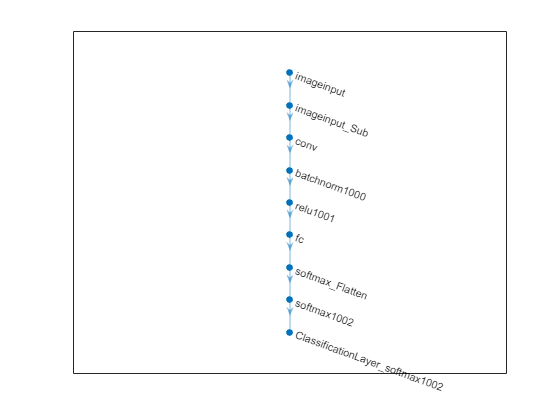Import a pretrained ONNX network as a LayerGraph object. Then, assemble the imported layers into a DAGNetwork object, and use the assembled network to classify an image.

Generate an ONNX model of the squeezenet convolution neural network.

squeezeNet = squeezenet;
exportONNXNetwork(squeezeNet,"squeezeNet.onnx");

Specify the model file and the class names.

modelfile = "squeezenet.onnx";
ClassNames = squeezeNet.Layers(end).Classes;

Import the layers and weights of the ONNX network. By default, importONNXLayers imports the network as a LayerGraph object compatible with a DAGNetwork object.

lgraph = importONNXLayers(modelfile)
lgraph =
LayerGraph with properties:

Layers: [70×1 nnet.cnn.layer.Layer]
Connections: [77×2 table]
InputNames: {'data'}
OutputNames: {'ClassificationLayer_prob'}

Analyze the imported network architecture.

analyzeNetwork(lgraph)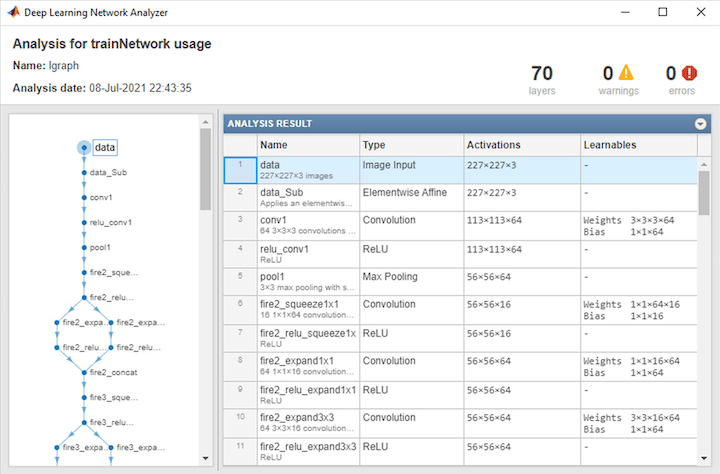Display the last layer of the imported network. The output shows that the layer graph has a ClassificationOutputLayer at the end of the network architecture.

lgraph.Layers(end)
ans =
ClassificationOutputLayer with properties:

Name: 'ClassificationLayer_prob'
Classes: 'auto'
ClassWeights: 'none'
OutputSize: 'auto'

Hyperparameters
LossFunction: 'crossentropyex'

The classification layer does not contain the classes, so you must specify these before assembling the network. If you do not specify the classes, then the software automatically sets the classes to 1, 2, ..., N, where N is the number of classes.

The classification layer has the name 'ClassificationLayer_prob'. Set the classes to ClassNames, and then replace the imported classification layer with the new one.

cLayer = lgraph.Layers(end);
cLayer.Classes = ClassNames;
lgraph = replaceLayer(lgraph,'ClassificationLayer_prob',cLayer);

Assemble the layer graph using assembleNetwork to return a DAGNetwork object.

net = assembleNetwork(lgraph)
net =
DAGNetwork with properties:

Layers: [70×1 nnet.cnn.layer.Layer]
Connections: [77×2 table]
InputNames: {'data'}
OutputNames: {'ClassificationLayer_prob'}

Read the image you want to classify and display the size of the image. The image is 384-by-512 pixels and has three color channels (RGB).

size(I)
ans = 1×3

384   512     3

Resize the image to the input size of the network. Show the image.

I = imresize(I,[227 227]);
imshow(I)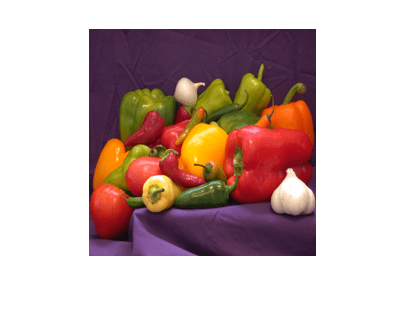Classify the image using the imported network.

label = classify(net,I)
label = categorical
bell pepper

Import a pretrained ONNX network as a LayerGraph object compatible with a dlnetwork object. Then, convert the layer graph to a dlnetwork to classify an image.

Generate an ONNX model of the squeezenet convolution neural network.

squeezeNet = squeezenet;
exportONNXNetwork(squeezeNet,"squeezeNet.onnx");

Specify the model file and the class names.

modelfile = "squeezenet.onnx";
ClassNames = squeezeNet.Layers(end).Classes;

Import the layers and weights of the ONNX network. Specify to import the network as a LayerGraph object compatible with a dlnetwork object.

lgraph = importONNXLayers(modelfile,TargetNetwork="dlnetwork")
lgraph =
LayerGraph with properties:

Layers: [70×1 nnet.cnn.layer.Layer]
Connections: [77×2 table]
InputNames: {'data'}
OutputNames: {1×0 cell}

Read the image you want to classify and display the size of the image. The image is 384-by-512 pixels and has three color channels (RGB).

size(I)
ans = 1×3

384   512     3

Resize the image to the input size of the network. Show the image.

I = imresize(I,[227 227]);
imshow(I)Convert the imported layer graph to a dlnetwork object.

dlnet = dlnetwork(lgraph);

Convert the image to a dlarray. Format the images with the dimensions "SSCB" (spatial, spatial, channel, batch). In this case, the batch size is 1 and you can omit it ("SSC").

I_dlarray = dlarray(single(I),"SSCB");

Classify the sample image and find the predicted label.

prob = predict(dlnet,I_dlarray);
[~,label] = max(prob);

Display the classification result.

ClassNames(label)
ans = categorical
bell pepper

Import a pretrained ONNX network as a LayerGraph object, and assemble the imported layers into a DAGNetwork object. Then, use the DAGNetwork to classify an image. The imported network contains ONNX operators that are not supported for conversion into built-in MATLAB layers. The software automatically generates custom layers when you import these operators.

This example uses the helper function findCustomLayers. To view the code for this function, see Helper Function.

Specify the file to import as shufflenet with operator set 9 from the ONNX Model Zoo. shufflenet is a convolutional neural network that is trained on more than a million images from the ImageNet database. As a result, the network has learned rich feature representations for a wide range of images. The network can classify images into 1000 object categories, such as keyboard, mouse, pencil, and many animals.

modelfile = "shufflenet-9.onnx";

Import the layers and weights of shufflenet. By default, importONNXLayers imports the network as a LayerGraph object compatible with a DAGNetwork object. If the imported network contains ONNX operators not supported for conversion into built-in MATLAB layers, then importONNXLayers can automatically generate custom layers in place of these layers. importONNXLayers saves each generated custom layer to a separate .m file in the package +shufflenet_9 in the current folder. Specify the package name by using the name-value argument PackageName.

lgraph = importONNXLayers(modelfile,PackageName="shufflenet_9")
lgraph =
LayerGraph with properties:

Layers: [173×1 nnet.cnn.layer.Layer]
Connections: [188×2 table]
InputNames: {'gpu_0_data_0'}
OutputNames: {'ClassificationLayer_gpu_0_softmax_1'}

Find the indices of the automatically generated custom layers by using the helper function findCustomLayers, and display the custom layers.

ind = findCustomLayers(lgraph.Layers,'+shufflenet_9');
lgraph.Layers(ind)
ans =
16×1 Layer array with layers:

1   'Reshape_To_ReshapeLayer1004'   shufflenet_9.Reshape_To_ReshapeLayer1004   shufflenet_9.Reshape_To_ReshapeLayer1004
2   'Reshape_To_ReshapeLayer1009'   shufflenet_9.Reshape_To_ReshapeLayer1009   shufflenet_9.Reshape_To_ReshapeLayer1009
3   'Reshape_To_ReshapeLayer1014'   shufflenet_9.Reshape_To_ReshapeLayer1014   shufflenet_9.Reshape_To_ReshapeLayer1014
4   'Reshape_To_ReshapeLayer1019'   shufflenet_9.Reshape_To_ReshapeLayer1019   shufflenet_9.Reshape_To_ReshapeLayer1019
5   'Reshape_To_ReshapeLayer1024'   shufflenet_9.Reshape_To_ReshapeLayer1024   shufflenet_9.Reshape_To_ReshapeLayer1024
6   'Reshape_To_ReshapeLayer1029'   shufflenet_9.Reshape_To_ReshapeLayer1029   shufflenet_9.Reshape_To_ReshapeLayer1029
7   'Reshape_To_ReshapeLayer1034'   shufflenet_9.Reshape_To_ReshapeLayer1034   shufflenet_9.Reshape_To_ReshapeLayer1034
8   'Reshape_To_ReshapeLayer1039'   shufflenet_9.Reshape_To_ReshapeLayer1039   shufflenet_9.Reshape_To_ReshapeLayer1039
9   'Reshape_To_ReshapeLayer1044'   shufflenet_9.Reshape_To_ReshapeLayer1044   shufflenet_9.Reshape_To_ReshapeLayer1044
10   'Reshape_To_ReshapeLayer1049'   shufflenet_9.Reshape_To_ReshapeLayer1049   shufflenet_9.Reshape_To_ReshapeLayer1049
11   'Reshape_To_ReshapeLayer1054'   shufflenet_9.Reshape_To_ReshapeLayer1054   shufflenet_9.Reshape_To_ReshapeLayer1054
12   'Reshape_To_ReshapeLayer1059'   shufflenet_9.Reshape_To_ReshapeLayer1059   shufflenet_9.Reshape_To_ReshapeLayer1059
13   'Reshape_To_ReshapeLayer1064'   shufflenet_9.Reshape_To_ReshapeLayer1064   shufflenet_9.Reshape_To_ReshapeLayer1064
14   'Reshape_To_ReshapeLayer1069'   shufflenet_9.Reshape_To_ReshapeLayer1069   shufflenet_9.Reshape_To_ReshapeLayer1069
15   'Reshape_To_ReshapeLayer1074'   shufflenet_9.Reshape_To_ReshapeLayer1074   shufflenet_9.Reshape_To_ReshapeLayer1074
16   'Reshape_To_ReshapeLayer1079'   shufflenet_9.Reshape_To_ReshapeLayer1079   shufflenet_9.Reshape_To_ReshapeLayer1079

The classification layer does not contain the classes, so you must specify these before assembling the network. If you do not specify the classes, then the software automatically sets the classes to 1, 2, ..., N, where N is the number of classes.

Import the class names from squeezenet, which is also trained with images from the ImageNet database.

SqueezeNet = squeezenet;
classNames = SqueezeNet.Layers(end).ClassNames;

The classification layer cLayer is the final layer of lgraph. Set the classes to classNames and then replace the imported classification layer with the new one.

cLayer = lgraph.Layers(end)
cLayer =
ClassificationOutputLayer with properties:

Name: 'ClassificationLayer_gpu_0_softmax_1'
Classes: 'auto'
ClassWeights: 'none'
OutputSize: 'auto'

Hyperparameters
LossFunction: 'crossentropyex'

cLayer.Classes = classNames;
lgraph = replaceLayer(lgraph,lgraph.Layers(end).Name,cLayer);

Assemble the layer graph using assembleNetwork. The function returns a DAGNetwork object that is ready to use for prediction.

net = assembleNetwork(lgraph)
net =
DAGNetwork with properties:

Layers: [173×1 nnet.cnn.layer.Layer]
Connections: [188×2 table]
InputNames: {'gpu_0_data_0'}
OutputNames: {'ClassificationLayer_gpu_0_softmax_1'}

Read the image you want to classify and display the size of the image. The image is 792-by-1056 pixels and has three color channels (RGB).

size(I)
ans = 1×3

792        1056           3

Resize the image to the input size of the network. Show the image.

I = imresize(I,[224 224]);
imshow(I)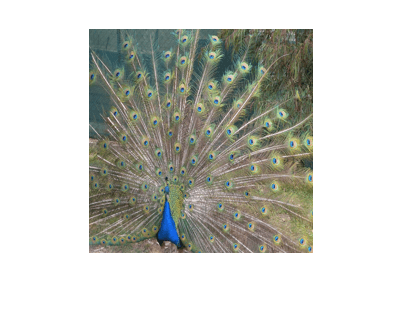The inputs to shufflenet require further preprocessing (for details, see ShuffleNet in ONNX Model Zoo). Rescale the image. Normalize the image by subtracting the mean of the training images and dividing by the standard deviation of the training images.

I = rescale(I,0,1);

meanIm = [0.485 0.456 0.406];
stdIm = [0.229 0.224 0.225];
I = (I - reshape(meanIm,[1 1 3]))./reshape(stdIm,[1 1 3]);

Classify the image using the imported network.

label = classify(net,I)
label = categorical
peacock

Helper Function

This section provides the code of the helper function findCustomLayers used in this example. findCustomLayers returns the indices of the custom layers that importONNXLayers automatically generates.

function indices = findCustomLayers(layers,PackageName)

s = what(['.\' PackageName]);

indices = zeros(1,length(s.m));
for i = 1:length(layers)
for j = 1:length(s.m)
if strcmpi(class(layers(i)),[PackageName(2:end) '.' s.m{j}(1:end-2)])
indices(j) = i;
end
end
end

end

Import an ONNX long short-term memory (LSTM) network as a layer graph, and then find and replace the placholder layers. An LSTM network enables you to input sequence data into a network, and make predictions based on the individual time steps of the sequence data.

lstmNet has a similar architecture to the LSTM network created in Sequence Classification Using Deep Learning. lstmNet is trained to recognize the speaker given time series data representing two Japanese vowels spoken in succession.

Specify lstmNet as the model file.

modelfile = "lstmNet.onnx";

Import the layers and weights of the ONNX network. By default, importONNXLayers imports the network as a LayerGraph object compatible with a DAGNetwork object.

lgraph = importONNXLayers("lstmNet.onnx")
Warning: Unable to import some ONNX operators, because they are not supported. They have been replaced by placeholder layers. To find these layers, call the function findPlaceholderLayers on the returned object.

1 operators(s)	:	Unable to create an output layer for the ONNX network output 'softmax1001' because its data format is unknown or unsupported by MATLAB output layers.
If you know its format, pass it using the 'OutputDataFormats' argument.

To import the ONNX network as a function, use importONNXFunction.
lgraph =
LayerGraph with properties:

Layers: [6×1 nnet.cnn.layer.Layer]
Connections: [5×2 table]
InputNames: {'sequenceinput'}
OutputNames: {1×0 cell}

importONNXLayers displays a warning and inserts a placeholder layer for the output layer.

You can check for placeholder layers by viewing the Layers property of lgraph or by using the findPlaceholderLayers function.

lgraph.Layers
ans =
6×1 Layer array with layers:

1   'sequenceinput'                 Sequence Input                        Sequence input with 12 dimensions
2   'lstm1000'                      LSTM                                  LSTM with 100 hidden units
3   'fc_MatMul'                     Fully Connected                       9 fully connected layer
4   'fc_Add'                        Elementwise Affine                    Applies an elementwise scaling followed by an addition to the input.
5   'Flatten_To_SoftmaxLayer1005'   lstmNet.Flatten_To_SoftmaxLayer1005   lstmNet.Flatten_To_SoftmaxLayer1005
6   'OutputLayer_softmax1001'       PLACEHOLDER LAYER                     Placeholder for 'added_outputLayer' ONNX operator
placeholderLayers = findPlaceholderLayers(lgraph)
placeholderLayers =
PlaceholderLayer with properties:

Name: 'OutputLayer_softmax1001'
ONNXNode: [1×1 struct]
Weights: []

Learnable Parameters
No properties.

State Parameters
No properties.

Show all properties

Create an output layer to replace the placeholder layer. First, create a classification layer with the name OutputLayer_softmax1001. If you do not specify the classes, then the software automatically sets them to 1, 2, ..., N, where N is the number of classes. In this case, the class data is a categorical vector of labels "1","2",..."9", which correspond to nine speakers.

outputLayer = classificationLayer('Name','OutputLayer_softmax1001');

Replace the placeholder layers with outputLayer by using the replaceLayer function.

lgraph = replaceLayer(lgraph,'OutputLayer_softmax1001',outputLayer);

Display the Layers property of the layer graph to confirm the replacement.

lgraph.Layers
ans =
6×1 Layer array with layers:

1   'sequenceinput'                 Sequence Input                        Sequence input with 12 dimensions
2   'lstm1000'                      LSTM                                  LSTM with 100 hidden units
3   'fc_MatMul'                     Fully Connected                       9 fully connected layer
4   'fc_Add'                        Elementwise Affine                    Applies an elementwise scaling followed by an addition to the input.
5   'Flatten_To_SoftmaxLayer1005'   lstmNet.Flatten_To_SoftmaxLayer1005   lstmNet.Flatten_To_SoftmaxLayer1005
6   'OutputLayer_softmax1001'       Classification Output                 crossentropyex

Alternatively, define the output layer when you import the layer graph by using the OutputLayerType or OutputDataFormats option. Check if the imported layer graphs have placeholder layers by using findPlaceholderLayers.

lgraph1 = importONNXLayers("lstmNet.onnx",OutputLayerType="classification");
findPlaceholderLayers(lgraph1)
ans =
0×1 Layer array with properties:

lgraph2 = importONNXLayers("lstmNet.onnx",OutputDataFormats="BC");
findPlaceholderLayers(lgraph2)
ans =
0×1 Layer array with properties:

The imported layer graphs lgraph1 and lgraph2 do not have placeholder layers.

Import an ONNX network that has multiple outputs by using importONNXLayers, and then assemble the imported layer graph into a DAGNetwork object.

Specify the network file from which to import layers and weights.

modelfile = "digitsMIMO.onnx";

Import the layers and weights from modelfile. The network in digitsMIMO.onnx has two output layers: one classification layer (ClassificationLayer_sm_1) to classify digits and one regression layer (RegressionLayer_fc_1_Flatten) to compute the mean squared error for the predicted angles of the digits.

lgraph = importONNXLayers(modelfile)
lgraph =
LayerGraph with properties:

Layers: [19×1 nnet.cnn.layer.Layer]
Connections: [19×2 table]
InputNames: {'input'}
OutputNames: {'ClassificationLayer_sm_1'  'RegressionLayer_fc_1_Flatten'}

Plot the layer graph using plot, and display the layers of lgraph.

plot(lgraph)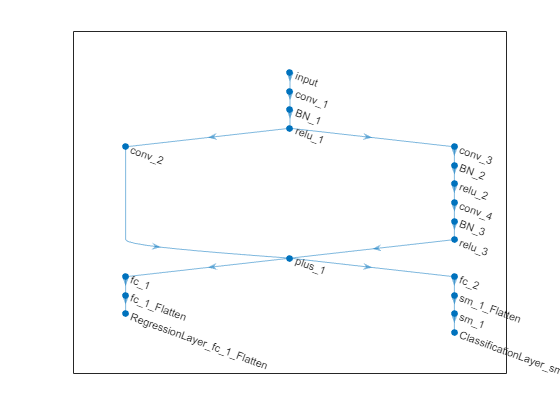lgraph.Layers
ans =
19×1 Layer array with layers:

1   'input'                          Image Input             28×28×1 images
2   'conv_1'                         Convolution             16 5×5×1 convolutions with stride [1  1] and padding [2  2  2  2]
3   'BN_1'                           Batch Normalization     Batch normalization with 16 channels
4   'relu_1'                         ReLU                    ReLU
5   'conv_2'                         Convolution             32 1×1×16 convolutions with stride [2  2] and padding [0  0  0  0]
6   'conv_3'                         Convolution             32 3×3×16 convolutions with stride [2  2] and padding [1  1  1  1]
7   'BN_2'                           Batch Normalization     Batch normalization with 32 channels
8   'relu_2'                         ReLU                    ReLU
9   'conv_4'                         Convolution             32 3×3×32 convolutions with stride [1  1] and padding [1  1  1  1]
10   'BN_3'                           Batch Normalization     Batch normalization with 32 channels
11   'relu_3'                         ReLU                    ReLU
13   'fc_1'                           Convolution             1 14×14×32 convolutions with stride [1  1] and padding [0  0  0  0]
14   'fc_2'                           Convolution             10 14×14×32 convolutions with stride [1  1] and padding [0  0  0  0]
15   'sm_1_Flatten'                   ONNX Flatten            Flatten activations into 1-D assuming C-style (row-major) order
16   'sm_1'                           Softmax                 softmax
17   'fc_1_Flatten'                   ONNX Flatten            Flatten activations into 1-D assuming C-style (row-major) order
18   'ClassificationLayer_sm_1'       Classification Output   crossentropyex
19   'RegressionLayer_fc_1_Flatten'   Regression Output       mean-squared-error

The classification layer does not contain the classes, so you must specify these before assembling the network. If you do not specify the classes, then the software automatically sets the classes to 1, 2, ..., N, where N is the number of classes. Specify the classes of cLayer as 0, 1, ..., 9. Then, replace the imported classification layer with the new one.

ClassNames = string(0:9);
cLayer = lgraph.Layers(18);
cLayer.Classes = ClassNames;
lgraph = replaceLayer(lgraph,"ClassificationLayer_sm_1",cLayer);

Assemble the layer graph using assembleNetwork. The function returns a DAGNetwork object that is ready to use for prediction.

assembledNet = assembleNetwork(lgraph)
assembledNet =
DAGNetwork with properties:

Layers: [19×1 nnet.cnn.layer.Layer]
Connections: [19×2 table]
InputNames: {'input'}
OutputNames: {'ClassificationLayer_sm_1'  'RegressionLayer_fc_1_Flatten'}

Input Arguments

collapse all

Name of the ONNX model file containing the network, specified as a character vector or string scalar. The file must be in the current folder or in a folder on the MATLAB path, or you must include a full or relative path to the file.

Example: "cifarResNet.onnx"

Name-Value Arguments

Specify optional pairs of arguments as Name1=Value1,...,NameN=ValueN, where Name is the argument name and Value is the corresponding value. Name-value arguments must appear after other arguments, but the order of the pairs does not matter.

Example: importONNXLayers(modelfile,TargetNetwork="dagnetwork",GenerateCustomLayers=true,PackageName="CustomLayers") imports the network layers from modelfile as a layer graph compatible with a DAGNetwork object and saves the automatically generated custom layers in the package +CustomLayers in the current folder.

Option for custom layer generation, specified as a numeric or logical 1 (true) or 0 (false). If you set GenerateCustomLayers to true, importONNXLayers tries to generate a custom layer when the software cannot convert an ONNX operator into an equivalent built-in MATLAB layer. importONNXLayers saves each generated custom layer to a separate .m file in +PackageName. To view or edit a custom layer, open the associated .m file. For more information on custom layers, see Deep Learning Custom Layers.

Example: GenerateCustomLayers=false

Name of the package in which importONNXLayers saves custom layers, specified as a character vector or string scalar. importONNXLayers saves the custom layers package +PackageName in the current folder. If you do not specify PackageName, then importONNXLayers saves the custom layers in a package named +modelfile in the current folder. For more information on packages, see Packages Create Namespaces.

Example: PackageName="shufflenet_9"

Example: PackageName="CustomLayers"

Target type of Deep Learning Toolbox network for imported network architecture, specified as "dagnetwork" or "dlnetwork". The function importONNXLayers imports the network architecture as a LayerGraph object compatible with a DAGNetwork or dlnetwork object.

• If you specify TargetNetwork as "dagnetwork", the imported lgraph must include input and output layers specified by the ONNX model or that you specify using the name-value arguments InputDataFormats, OutputDataFormats, or OutputLayerType.

• If you specify TargetNetwork as "dlnetwork", importONNXLayers appends a CustomOutputLayer at the end of each output branch of lgraph, and might append a CustomInputLayer at the beginning of an input branch. The function appends a CustomInputLayer if the input data formats or input image sizes are not known. For network-specific information on the data formats of these layers, see the properties of the CustomInputLayer and CustomOutputLayer objects. For information on how to interpret Deep Learning Toolbox input and output data formats, see Conversion of ONNX Input and Output Tensors into Built-In MATLAB Layers.

Example: TargetNetwork="dlnetwork" imports a LayerGraph object compatible with a dlnetwork object.

Data format of the network inputs, specified as a character vector, string scalar, or string array. importONNXLayers tries to interpret the input data formats from the ONNX file. The name-value argument InputDataFormats is useful when importONNXLayers cannot derive the input data formats.

Set InputDataFomats to a data format in the ordering of an ONNX input tensor. For example, if you specify InputDataFormats as "BSSC", the imported network has one imageInputLayer input. For more information on how importONNXLayers interprets the data format of ONNX input tensors and how to specify InputDataFormats for different Deep Learning Toolbox input layers, see Conversion of ONNX Input and Output Tensors into Built-In MATLAB Layers.

If you specify an empty data format ([] or ""), importONNXLayers automatically interprets the input data format.

Example: InputDataFormats='BSSC'

Example: InputDataFormats="BSSC"

Example: InputDataFormats=["BCSS","","BC"]

Example: InputDataFormats={'BCSS',[],'BC'}

Data Types: char | string | cell

Data format of the network outputs, specified as a character vector, string scalar, or string array. importONNXLayers tries to interpret the output data formats from the ONNX file. The name-value argument OutputDataFormats is useful when importONNXLayers cannot derive the output data formats.

Set OutputDataFormats to a data format in the ordering of an ONNX output tensor. For example, if you specify OutputDataFormats as "BC", the imported network has one classificationLayer output. For more information on how importONNXLayers interprets the data format of ONNX output tensors and how to specify OutputDataFormats for different Deep Learning Toolbox output layers, see Conversion of ONNX Input and Output Tensors into Built-In MATLAB Layers.

If you specify an empty data format ([] or ""), importONNXLayers automatically interprets the output data format.

Example: OutputDataFormats='BC'

Example: OutputDataFormats="BC"

Example: OutputDataFormats=["BCSS","","BC"]

Example: OutputDataFormats={'BCSS',[],'BC'}

Data Types: char | string | cell

Size of the input image for the first network input, specified as a vector of three or four numerical values corresponding to [height,width,channels] for 2-D images and [height,width,depth,channels] for 3-D images. The network uses this information only when the ONNX model in modelfile does not specify the input size.

Example: ImageInputSize=[28 28 1] for a 2-D grayscale input image

Example: ImageInputSize=[224 224 3] for a 2-D color input image

Example: ImageInputSize=[28 28 36 3] for a 3-D color input image

Layer type for the first network output, specified as "classification", "regression", or "pixelclassification". The function importONNXLayers appends a ClassificationOutputLayer, RegressionOutputLayer, or pixelClassificationLayer (Computer Vision Toolbox) object to the end of the first output branch of the imported network architecture. Appending a pixelClassificationLayer (Computer Vision Toolbox) object requires Computer Vision Toolbox™. If the ONNX model in modelfile specifies the output layer type or you specify TargetNetwork as "dlnetwork", importONNXLayers ignores the name-value argument OutputLayerType.

Example: OutputLayerType="regression"

Constant folding optimization, specified as "deep", "shallow", or "none". Constant folding optimizes the imported network architecture by computing operations on ONNX initializers (initial constant values) during the conversion of ONNX operators to equivalent built-in MATLAB layers.

If the ONNX network contains operators that the software cannot convert to equivalent built-in MATLAB layers (see ONNX Operators Supported for Conversion into Built-In MATLAB Layers), then importONNXLayers inserts a placeholder layer in place of each unsupported layer. For more information, see Tips.

Constant folding optimization can reduce the number of placeholder layers. When you set FoldConstants to "deep", the imported layers include the same or fewer placeholder layers, compared to when you set the argument to "shallow". However, the importing time might increase. Set FoldConstants to "none" to disable the network architecture optimization.

Example: FoldConstants="shallow"

Output Arguments

collapse all

Network architecture of the pretrained ONNX model, returned as a LayerGraph object.

To use the imported layer graph for prediction, you must convert the LayerGraph object to a DAGNetwork or dlnetwork object. Specify the name-value argument TargetNetwork as "dagnetwork" or "dlnetwork" depending on the intended workflow.

• Convert a LayerGraph to a DAGNetwork by using assembleNetwork. On the DAGNetwork object, you then predict class labels using the classify function.

• Convert a LayerGraph to a dlnetwork by using dlnetwork. On the dlnetwork object, you then predict class labels using the predict function. Specify the input data as a dlarray using the correct data format (for more information, see the fmt argument of dlarray).

Limitations

• importONNXLayers supports ONNX versions as follows:

• The function supports ONNX intermediate representation version 6.

• The function supports ONNX operator sets 6 to 13.

Note

If you import an exported network, layers of the reimported network might differ from the original network and might not be supported.

collapse all

ONNX Operators Supported for Conversion into Built-In MATLAB Layers

importONNXLayers supports the following ONNX operators for conversion into built-in MATLAB layers, with some limitations.

ONNX OperatorDeep Learning Toolbox Layer

AveragePool

averagePooling2dLayer

BatchNormalization

batchNormalizationLayer

Concat

concatenationLayer

Constant

None (Imported as weights)

Conv*

convolution2dLayer

ConvTranspose

transposedConv2dLayer

Dropout

dropoutLayer

Elu

eluLayer

Gemm

fullyConnectedLayer if ONNX network is recurrent, otherwise nnet.onnx.layer.FlattenLayer followed by convolution2dLayer

GlobalAveragePool

globalAveragePooling2dLayer

GlobalMaxPool

globalMaxPooling2dLayer

GRU

gruLayer

InstanceNormalization

groupNormalizationLayer with numGroups specified as "channel-wise"

LeakyRelu

leakyReluLayer

LRN

CrossChannelNormalizationLayer

LSTM

lstmLayer or bilstmLayer

MatMul

fullyConnectedLayer if ONNX network is recurrent, otherwise convolution2dLayer

MaxPool

maxPooling2dLayer

Mul

multiplicationLayer

Relu

reluLayer or clippedReluLayer

Sigmoid

sigmoidLayer

Softmax

softmaxLayer

Sum

Tanh

tanhLayer

*If the pads attribute of the Conv operator is a vector with only two elements [p1,p2], importONNXLayers imports Conv as a convolution2dLayer with the name-value argument 'Padding' specified as [p1,p2,p1,p2].

ONNX OperatorONNX Importer Custom Layer

Clip

nnet.onnx.layer.ClipLayer

Div

nnet.onnx.layer.ElementwiseAffineLayer

Flatten

nnet.onnx.layer.FlattenLayer or nnet.onnx.layer.Flatten3dLayer

Identity

nnet.onnx.layer.IdentityLayer

ImageScaler

nnet.onnx.layer.ElementwiseAffineLayer

PRelu

nnet.onnx.layer.PReluLayer

Reshape

nnet.onnx.layer.FlattenLayer

Sub

nnet.onnx.layer.ElementwiseAffineLayer
ONNX OperatorImage Processing Toolbox™
DepthToSpacedepthToSpace2dLayer (Image Processing Toolbox)
Resizeresize2dLayer (Image Processing Toolbox) or resize3dLayer (Image Processing Toolbox)
SpaceToDepthspaceToDepthLayer (Image Processing Toolbox)
Upsampleresize2dLayer (Image Processing Toolbox) or resize3dLayer (Image Processing Toolbox)

Conversion of ONNX Input and Output Tensors into Built-In MATLAB Layers

importONNXLayers tries to interpret the data format of the ONNX network's input and output tensors, and then convert them into built-in MATLAB input and output layers. For details on the interpretation, see the tables Conversion of ONNX Input Tensors into Deep Learning Toolbox Layers and Conversion of ONNX Output Tensors into MATLAB Layers.

In Deep Learning Toolbox, each data format character must be one of these labels:

• S — Spatial

• C — Channel

• B — Batch observations

• T — Time or sequence

• U — Unspecified

Conversion of ONNX Input Tensors into Deep Learning Toolbox Layers

Data FormatsData InterpretationDeep Learning Toolbox Layer
ONNX Input Tensor MATLAB Input FormatShapeType
BCCBc-by-n array, where c is the number of features and n is the number of observationsFeaturesfeatureInputLayer
BCSS, BSSC, CSS, SSCSSCB

h-by-w-by-c-by-n numeric array, where h, w, c and n are the height, width, number of channels of the images, and number of observations, respectively

2-D imageimageInputLayer
BCSSS, BSSSC, CSSS, SSSCSSSCB

h-by-w-by-d-by-c numeric array, where h, w, d, c and n are the height, width, depth, number of channels of the images, and number of image observations, respectively

3-D imageimage3dInputLayer
TBCCBT

c-by-s-by-n matrix, where c is the number of features of the sequence, s is the sequence length, and n is the number of sequence observations

Vector sequencesequenceInputLayer
TBCSSSSCBT

h-by-w-by-c-by-s-by-n array, where h, w, c and n correspond to the height, width, and number of channels of the image, respectively, s is the sequence length, and n is the number of image sequence observations

2-D image sequencesequenceInputLayer
TBCSSSSSSCBT

h-by-w-by-d-by-c-by-s-by-n array, where h, w, d, and c correspond to the height, width, depth, and number of channels of the image, respectively, s is the sequence length, and n is the number of image sequence observations

3-D image sequencesequenceInputLayer

Conversion of ONNX Output Tensors into MATLAB Layers

Data FormatsMATLAB Layer
ONNX Output TensorMATLAB Output Format
BC, TBCCB, CBTclassificationLayer
BCSS, BSSC, CSS, SSC, BCSSS, BSSSC, CSSS, SSSCSSCB, SSSCBpixelClassificationLayer (Computer Vision Toolbox)
TBCSS, TBCSSSSSCBT, SSSCBTregressionLayer

Use Imported Network Layers on GPU

importONNXLayers does not execute on a GPU. However, importONNXLayers imports the layers of a pretrained neural network for deep learning as a LayerGraph object, which you can use on a GPU.

• Convert the imported LayerGraph object to a DAGNetwork object by using assembleNetwork. On the DAGNetwork object, you can then predict class labels on either a CPU or GPU by using classify. Specify the hardware requirements using the name-value argument ExecutionEnvironment. For networks with multiple outputs, use the predict function and specify the name-value argument ReturnCategorical as true.

• Convert the imported LayerGraph object to a dlnetwork object by using dlnetwork. On the dlnetwork object, you can then predict class labels on either a CPU or GPU by using predict. The function predict executes on the GPU if either the input data or network parameters are stored on the GPU.

• If you use minibatchqueue to process and manage the mini-batches of input data, the minibatchqueue object converts the output to a GPU array by default if a GPU is available.

• Use dlupdate to convert the learnable parameters of a dlnetwork object to GPU arrays.

dlnet = dlupdate(@gpuarray,dlnet)

• You can train the imported LayerGraph object on either a CPU or GPU by using trainNetwork. To specify training options, including options for the execution environment, use the trainingOptions function. Specify the hardware requirements using the name-value argument ExecutionEnvironment. For more information on how to accelerate training, see Scale Up Deep Learning in Parallel, on GPUs, and in the Cloud.

Using a GPU requires Parallel Computing Toolbox™ and a supported GPU device. For information on supported devices, see GPU Support by Release (Parallel Computing Toolbox).

Tips

• If the imported network contains an ONNX operator not supported for conversion into a built-in MATLAB layer (see ONNX Operators Supported for Conversion into Built-In MATLAB Layers) and importONNXLayers does not generate a custom layer, then importONNXLayers inserts a placeholder layer in place of the unsupported layer. To find the names and indices of the unsupported layers in the network, use the findPlaceholderLayers function. You then can replace a placeholder layer with a new layer that you define. To replace a layer, use replaceLayer. For an example, see Import and Assemble ONNX Network with Multiple Outputs.

• To use a pretrained network for prediction or transfer learning on new images, you must preprocess your images in the same way the images that were used to train the imported model were preprocessed. The most common preprocessing steps are resizing images, subtracting image average values, and converting the images from BGR images to RGB.

• To resize images, use imresize. For example, imresize(image,[227,227,3]).

• To convert images from RGB to BGR format, use flip. For example, flip(image,3).

For more information on preprocessing images for training and prediction, see Preprocess Images for Deep Learning.

Alternative Functionality

Deep Learning Toolbox Converter for ONNX Model Format provides three functions to import a pretrained ONNX network: importONNXNetwork, importONNXLayers, and importONNXFunction. For more information on which import function best suits different scenarios, see Select Function to Import ONNX Pretrained Network.

Compatibility Considerations

expand all

Warns starting in R2021b

Behavior changed in R2021b

 Open Neural Network Exchange. https://github.com/onnx/.

 ONNX. https://onnx.ai/.